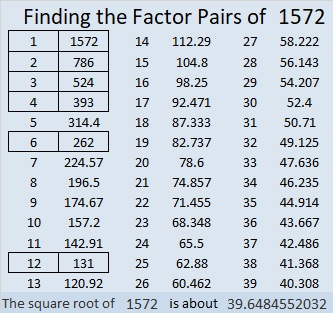# 1572 Twelve Orientations of a Magic Snowflake

Contents

### Today’s Puzzle:

Without looking, can you decide where the numbers 1 to 13 go on a snowflake so that each of the six edges and each of the six lines of symmetry has the same sum? There is only one solution, but the snowflake can be put into twelve different orientations. Perhaps you solved that puzzle by writing in the numbers when I suggested it a few days ago. You will want to solve that puzzle first.

The twelve orientations are pictured below. They are all pictures of the same snowflake.Making a six-sided snowflake is more complicated than making a four-sided one. Rather than give step by step pictures of how I made the snowflake, I’ll just show one picture and explain the steps.1. Fold a piece of printer paper along a diagonal, cut off the excess to form a square.
2. Find the center of that diagonal and divide the folded paper into thirds that intersect the center. Cut the raw edge to make the largest possible equilateral triangle that is 6-sheets thick. Unfold. You now have a hexagon.
3. Fold an edge of the hexagon to the center but only make the crease go from one line of symmetry to the next one. Unfold. Repeat until all six edges have been folded to the center. This will create a medium-size hexagon inside the bigger hexagon.
4. Fold back into a 6-sheet-thick equilateral triangle. Divide the cut side into thirds and fold at a 90-degree angle to the cut side. Only crease from the edge to the medium-size hexagon. Unfold.
5. Cut along the fold you just made to create small slits in each of the twelve creases.
6. Cut a small slit along the folds of each side of the medium-size hexagon.
7. Fold back into a 6-sheet-thick equilateral triangle. Use decorative scissors to cut along the edge of the cut side of the triangle to make a pretty edge for the snowflake. Unfold until the only fold is along the diagonal.
8. Using a hole punch but only going halfway on the paper with the punch, make the center punch. It will unfold into a single punch. Fold back into a 6-sheet-thick equilateral triangle. Make a punch through all 6 sheets but keep the punch very close to the center punch. This will create the 7-punch small hexagon in the center of the snowflake.
9. Using the hole punch and any necessary creases, create hole punches representing the other numbers from 1 to 13. Take care that every edge and every line of symmetry has the same sum.

### Factors of 1572:

• 1572 is a composite number.
• Prime factorization: 1572 = 2 × 2 × 3 × 131, which can be written 1572 = 2² × 3 × 131.
• 1572 has at least one exponent greater than 1 in its prime factorization so √1572 can be simplified. Taking the factor pair from the factor pair table below with the largest square number factor, we get √1572 = (√4)(√393) = 2√393.
• The exponents in the prime factorization are 2, 1, and 1. Adding one to each exponent and multiplying we get (2 + 1)(1 + 1)(1 + 1) = 3 × 2 × 2 = 12. Therefore 1572 has exactly 12 factors.
• The factors of 1572 are outlined with their factor pair partners in the graphic below.### More about the Number 1572:

1572 is the difference of two squares two different ways:
394² – 392² = 1572 and
134² – 128² = 1572.

Since 1572 is divisible by 3, it is the magic sum of a magic hexagon. There isn’t room to punch enough holes in the hexagon, but you can see where all the numbers go below.This site uses Akismet to reduce spam. Learn how your comment data is processed.